Function Repository Resource:

# AberrancyCurve

Compute the curve of aberrancy of a plane curve

Contributed by: Jan Mangaldan
 ResourceFunction["AberrancyCurve"][c,t] computes the curve of aberrancy of the plane curve c parametrized by variable t.

## Details

The curve of aberrancy is also known as the affine evolute.
The curve of aberrancy is the envelope of the lines that are parallel to the axes of a plane curve's osculating parabolas and pass through their point of contact with the plane curve.
The curve of aberrancy is the locus of the centers of aberrancy of a plane curve.

## Examples

### Basic Examples (2)

Define the parametric equations for an astroid:

 In:=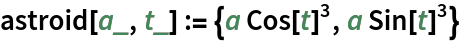Compute its curve of aberrancy:

 In:=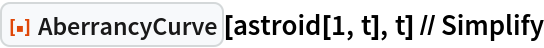Out=Plot the astroid and its curve of aberrancy:

 In:=Out=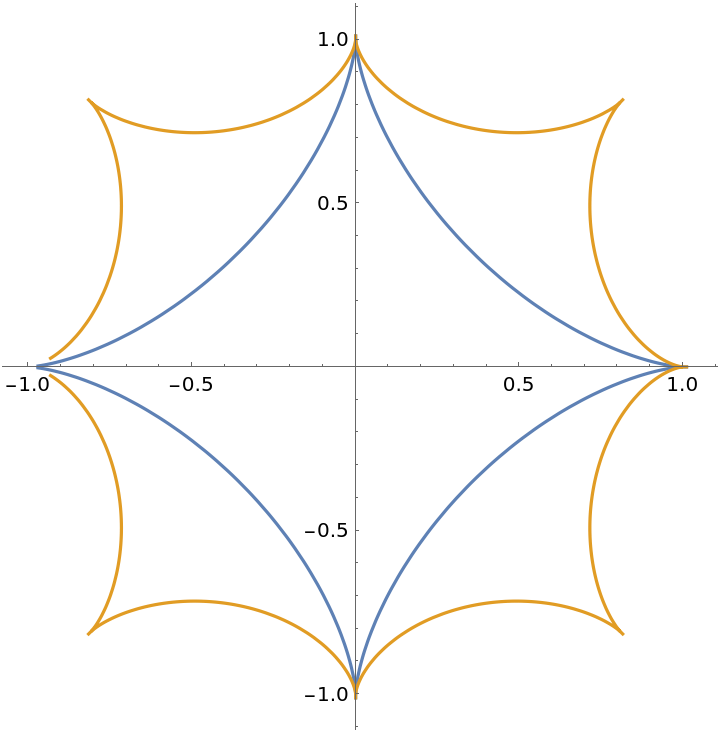Compute the implicit equation for the curve of aberrancy of a quartic:

 In:=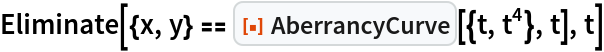Out=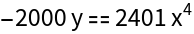### Properties and Relations (1)

The curve of aberrancy of a plane curve involves third and fourth derivatives:

 In:=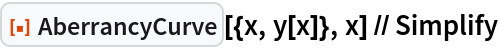Out=## Version History

• 1.0.0 – 04 March 2021